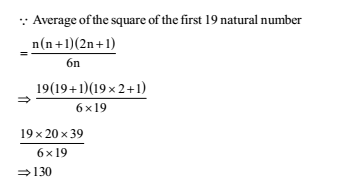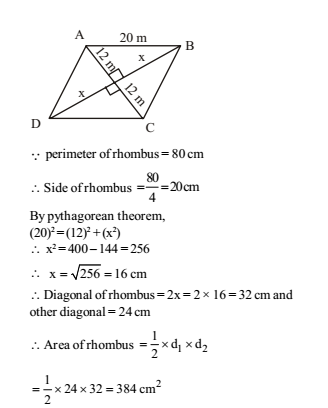# RRB ALP 2018 Practice Test Papers | Arithmetic Questions (Day-36)

Dear Aspirants, Here we have given the Important RRB ALP & Technicians Exam 2018 Practice Test Papers. Candidates those who are preparing for RRB ALP 2018 can practice these Arithmetic Questions to get more confidence to Crack RRB 2018 Examination.

[WpProQuiz 2103]

Click “Start Quiz” to attend these Questions and view Explanation

1. In a match, average of runs scored by 5 players is 49. If the runs scored by four players are 75, 30, 62 and 21 respectively, then how many runs did the 5th player had scored?

(a) 43

(b) 49

(c) 57

(d) 89

1. Raman, Manan, and Kamal are partners and invest in a business such that Raman invests 2/5th of total and Manan invest 3/8th of the total. What is the ratio of profit of Raman, Manan and Kamal respectively?

(a) 16: 15: 9

(b) 16: 15: 31

(c) 2: 3: 5

(d) 15: 16: 9

3.A train 150 m long passes a km stone in 30 seconds and another train of the same length travelling in opposite direction in 10 seconds. The speed of the second train is:

(a) 125 km/hr

(b) 25 km/hr

(c) 90 km/hr

(d) 75 km/hr

1. If water is freezed to become ice, its volume is increased by 10%, then if the ice is melted to water again, its volume will be decreased by:

(a) 8%

(b) 9 ½ %

(c) 9%

(d) 9 1/ 11 %

1. If x * y = (x + 3)2 (y –1), then the value of 5 * 4 is

(a) 192

(b) 182

(c) 2

(d) 356

1. A number of boys raised Rs.12,544 for a famine fund, each boy has given as many rupees as there were boys. The number of boys was:

(a) 122

(b) 132

(c) 112

(d) 102

1. What is the average of the squares of the first 19 natural numbers?

(a) 124

(b) 127.5

(c) 130

(d) 133.5

1. If the perimeter of a rhombus is 80 cm and one of its diagonal is 24 cm, then what is the area (in cm2) of the rhombus?

(a) 384

(b) 192

(c) 218

(d) 768

1. The average of 13 results is 70. The average of first seven is 65 and that of the last seven is 75, the seventh result is:

(a) 70

(b) 70.5

(c) 68

(d) 67

1. The length of two parallel sides of a trapezium are 15 cm and 20 cm. If its area is 175 sq. cm, then its height is:

(a) 10 cm

(b) 15 cm

(c) 25 cm

(d) 20 cm

Total runs scored by 5 players = (49 × 5) = 245

Total runs scored by 4 players = (75 + 30 + 62 + 21) = 188

Total runs did the 5th player had scored = (245 – 188) = 57.

Let total investment = x

Investment by Raman= 2x/ 5

Investment by Manan =3x/ 8

Investment by Kamal =x-(2x/5+3x/8)9x 40

Ratio of invest of Raman, Manan and Kamal= 2x/5: 3x/8: 9x/40 = 16 :15: 9

Ratio of profit of Raman, Manan and Kamal = 16: 15: 9

Speed of first train = 150 30 =5m/sec

Let the speed of second train be x m/sec

Relative speed = (5 + x) m /sec

300 /(5+ x) = 10

50 + 10x = 300

x = (300 -50 )/10 = 25m/sec

= 25 × 18/ 5 = 90km/h

Let initial volume = 100

Volume after increase = 100× 110 /100 = 110

So, decrease =( 110 -100 )/110 ×100 = 10/ 110 x100  = 9  1/ 11%

x * y = (x + 3)2 (y – 1)

5 * 4 = (5 + 3)2 (4 – 1) = 64 × 3 = 192

Contribution of each boy = Number of boys Total contribution raised = Rs. 12544

So, number of boys = √12544 = 112

1. Answer: (c)Sum of 13 results = 13 × 70 = 910

Sum of 7 results = 7 × 65 = 455

Sum of last 7 results = 7 × 75 = 525

So, 7th result (455 + 525) – 910 = 70

Area of trapezium = Sum of length of parallel sides/2 x Height (H)

175 =( 15 +20)/2  ×H

H = 175x 2 /35= 10cm.

RRB ALP 2018 Practice Test Papers | Arithmetic Questions (Day-1)

RRB ALP 2018 Practice Test Papers | Arithmetic Questions (Day-2)

RRB ALP 2018 Practice Test Papers | Arithmetic Questions (Day-3)

RRB ALP 2018 Practice Test Papers | Arithmetic Questions (Day-4)

RRB ALP 2018 Practice Test Papers | Arithmetic Questions (Day-5)

RRB ALP 2018 Practice Test Papers | Arithmetic Questions (Day-6)

RRB ALP 2018 Practice Test Papers | Arithmetic Questions (Day-7)

RRB ALP 2018 Practice Test Papers | Arithmetic Questions (Day-8)

RRB ALP 2018 Practice Test Papers | Arithmetic Questions (Day-9)

RRB ALP 2018 Practice Test Papers | Arithmetic Questions (Day-10)

RRB ALP 2018 Practice Test Papers | Arithmetic Questions (Day-11)

RRB ALP 2018 Practice Test Papers | Arithmetic Questions (Day-12)

RRB ALP 2018 Practice Test Papers | Arithmetic Questions (Day-13)

RRB ALP 2018 Practice Test Papers | Arithmetic Questions (Day-14)

RRB ALP 2018 Practice Test Papers | Arithmetic Questions (Day-15)

RRB ALP 2018 Practice Test Papers | Arithmetic Questions (Day-16)

RRB ALP 2018 Practice Test Papers | Arithmetic Questions (Day-17)

RRB ALP 2018 Practice Test Papers | Arithmetic Questions (Day-18)

RRB ALP 2018 Practice Test Papers | Arithmetic Questions (Day-19)

RRB ALP 2018 Practice Test Papers | Arithmetic Questions (Day-20)

RRB ALP 2018 Practice Test Papers | Arithmetic Questions (Day-21)

RRB ALP 2018 Practice Test Papers | Arithmetic Questions (Day-22)

RRB ALP 2018 Practice Test Papers | Arithmetic Questions (Day-23)

RRB ALP 2018 Practice Test Papers | Arithmetic Questions (Day-24)

RRB ALP 2018 Practice Test Papers | Arithmetic Questions (Day-25)

RRB ALP 2018 Practice Test Papers | Arithmetic Questions (Day-26)

RRB ALP 2018 Practice Test Papers | Arithmetic Questions (Day-27)

RRB ALP 2018 Practice Test Papers | Arithmetic Questions (Day-28)

RRB ALP 2018 Practice Test Papers | Arithmetic Questions (Day-29)

RRB ALP 2018 Practice Test Papers | Arithmetic Questions (Day-30)

RRB ALP 2018 Practice Test Papers | Arithmetic Questions (Day-31)

RRB ALP 2018 Practice Test Papers | Arithmetic Questions (Day-32)

RRB ALP 2018 Practice Test Papers | Arithmetic Questions (Day-33)

RRB ALP 2018 Practice Test Papers | Arithmetic Questions (Day-34)

RRB ALP 2018 Practice Test Papers | Arithmetic Questions (Day-35)